## Wednesday, September 14, 2016

### It's a small world, scale-free network after all

Real social networks generally have the properties of small world graphs (high clustering and low path lengths) and the characteristics of scale free networks (a heavy-tailed degree distribution).

The Watts-Strogatz (WS) network model has small world characteristics, but the degree distribution is roughly normal, very different from observed distributions.

The Barabasi-Albert (BA) model has low path lengths and a heavy-tailed degree distribution, but

1. It has low clustering, and
2. The degree distribution does not fit observed data well.

The Holmes-Kim (HK) model generates graphs with higher clustering, although still not as high as observed values. And the degree distribution is heavy tailed, but it still doesn't fit observed distributions well.

I propose a new model that generates graphs with

1. Low path lenths,
2. Clustering coefficients similar to the HK model (but still lower than observed values), and
3. A degree distribution that fits observed data well.

I test the models with a relatively small dataset from SNAP.

The proposed model is based on a "friend of a friend" growth mechanism that is a plausible description of the way social networks actually grow. The implementation is simple, comparable to BA and HK in both lines of code and run time.

All the details are in this Jupyter notebook, but I summarize the primary results here.

### Comparing the models

The Facebook dataset from SNAP contains 4039 nodes and 88234 edges.  The mean path length is 3.7 and the clustering coefficient is 0.6.

A WS model with the same number of nodes and edges, and with probability of rewiring, p=0.05, has mean path length 3.2 and clustering 0.62, so it clearly has the small world properties.  But the distribution of degree does not match the data at all: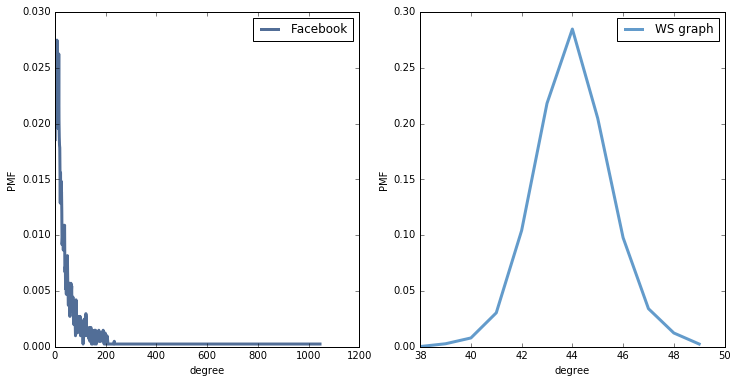A BA model with the same number of nodes and edges has very short paths (2.5), but very low clustering (0.04).  The degree distribution is a better match for the data: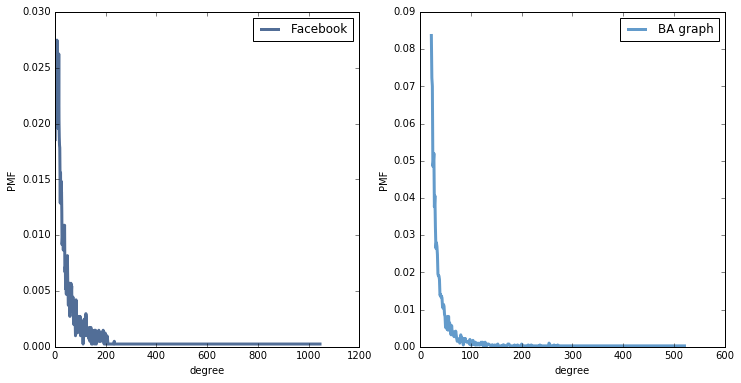If we plot CDFs on a log-log scale, the BA model matches the tail of the distribution reasonably well, but the WS model is hopeless.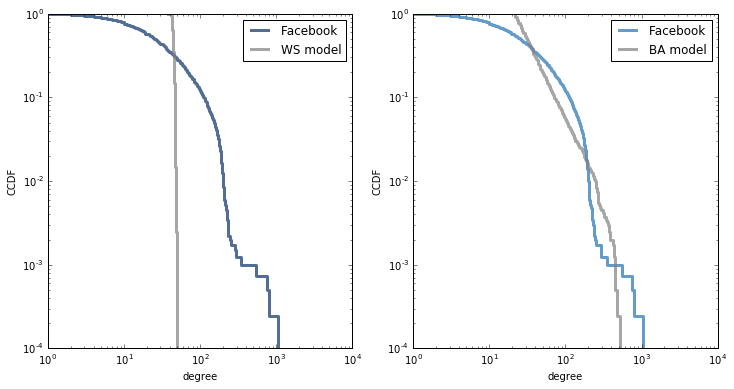But if we plot CDFs on a log-x scale, we see that the BA model does not match the rest of the distribution: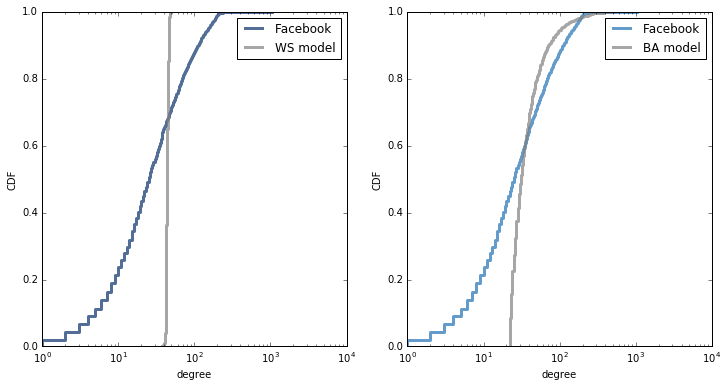The HK model also has short path lengths (2.8), and the clustering is much better (0.23), but still not as high as in the data (0.6).  The degree distribution is pretty much the same as in the BA model.

The FOF model

The generative model I propose is called FOF for "friends of friends". It is similar to both BA and HK, but it yields a degree distribution that matches observed data better.

It starts with a complete graph with m nodes, so initially all nodes have degree m. Each time we generate a node we:

1. Select a random target uniformly from existing nodes.
2. Iterate through the friends of the target. For each one, with probability p, we form a triangle that includes the source, friend, and a random friend of friend.
3. Finally, we connect the source and target.

Because we choose friends of the target, this process has preferential attachment, but it does not yield a power law tail. Rather, the degree distribution is approximately lognormal with median degree m.
Because this process forms triangles, it yields a moderately high clustering coefficient.

A FOF graph with the same number of nodes and edges as the Facebook data has low path length (3.0) and moderate clustering (0.24, which is more than BA, comparable to HK, but still less than the observed value, 0.6).

The degree distribution is a reasonable match for the tail of the observed distribution: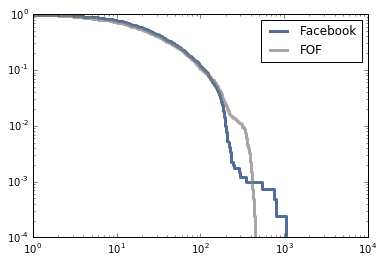And a good match for the rest of the distribution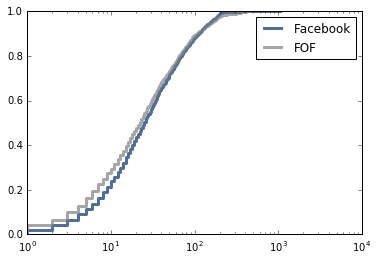In summary, the FOF model has

• Short path lengths, like WS, BA, and HK.
• Moderate clustering, similar to HK, less than WS, and higher than BA.
• Good fit to the tail of the degree distribution, like BA and HK.
• Good fit to the rest of the degree distribution, unlike WS, BA, and HK.

Also, the mechanism of growth is plausible: when a person joins the network, they connect to a randomly-chosen friend and then a random subset of "friends of friends". This process has preferential attachment because friends of friends are more likely to have high degree (see The Inspection Paradox is Everywhere) But the resulting distribution is approximately lognormal, which is heavy tailed, but does not have a power law tail.

### Implementation

Here is a function that generates FOF graphs:

def fof_graph(n, m, p=0.25, seed=None):
if m < 1 or  m+1 >= n:
raise nx.NetworkXError()
if seed is not None:
random.seed(seed)

G = nx.complete_graph(m+1)

for source in range(len(G), n):
# choose a random node
target = random.choice(G.nodes())

# enumerate neighbors of target and add triangles
friends = G.neighbors(target)
k = len(friends)
for friend in friends:
if flip(p):
triangle(G, source, friend)

# connect source and target

return G

def flip(p):
return random.random() < p

def triangle(G, source, friend):
"""Chooses a random neighbor of `friend` and makes a triangle.

Triangle connects `source`, `friend`, and
random neighbor of `friend`.
"""
fof = set(G[friend])
if source in G:
fof -= set(G[source])
if fof:
w = random.choice(list(fof))

Again, all the details are in this Jupyter notebook.

1.I enjoyed this blog post very much. I didn't think about scale free networks for some years. However, I see some waek points:
- the only parameter that alows you to controll the degree sequence and the clustering coefficient independently of the average degree is the rewiring probability. this means that the clustering coefficient and the distribution do strongly dependend on each other.
-every edge is part of a triangle.

There is a the model of hyperbolic randomg raphs which became very popular in the last few years. Its possible to control its clustering, average degree and degree sequence. The degree sequence can be power law distributed, the clustering can be strong and the average degree can be small. The model was introduced here:
http://arxiv.org/pdf/1006.5169.pdf
Later we analyzed it rigorously here: http://arxiv.org/pdf/1205.1470.pdf

Since then many other usefull properties like small average path lengh, efficient greedy routing etc. have been proven. Moreover, on a phylosophical level, it give (in my opinion) a very nice explanation for the properties of social networks, the internet graphs and other small world network.

1.